# 3-Digit Column Subtraction with Borrowing / Regrouping

3-Digit Column Subtraction with Borrowing / Regrouping – Maths with Mum

Supporting Lessons

## Column Subtraction with Borrowing / Regrouping: Worksheets and Answers

Download our printable workbooks for further practice of column subtraction with Borrowing / Regrouping!

## How to do Column Subtraction with Borrowing / Regrouping

In order to calculate the column subtraction of 592 – 226, we must begin by lining up the numbers so that their digits are in the correct place value columns.

We want to subtract 226, so this must be written below 592.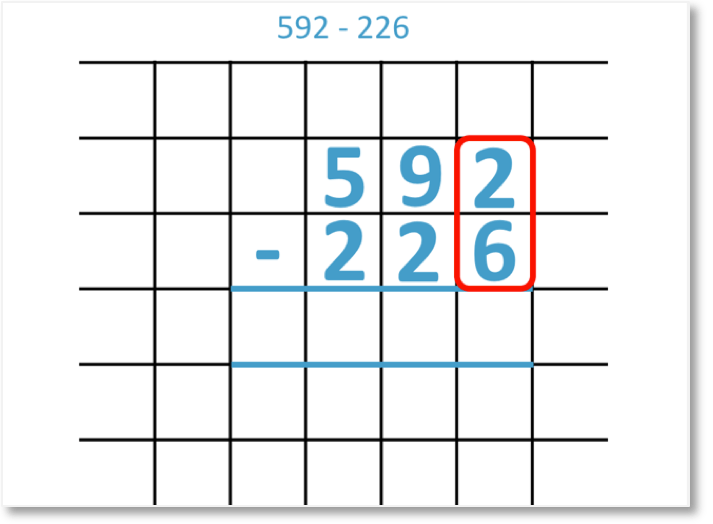We begin with the units column. However, the 6 is larger than the 2, so we can’t subtract it without regrouping.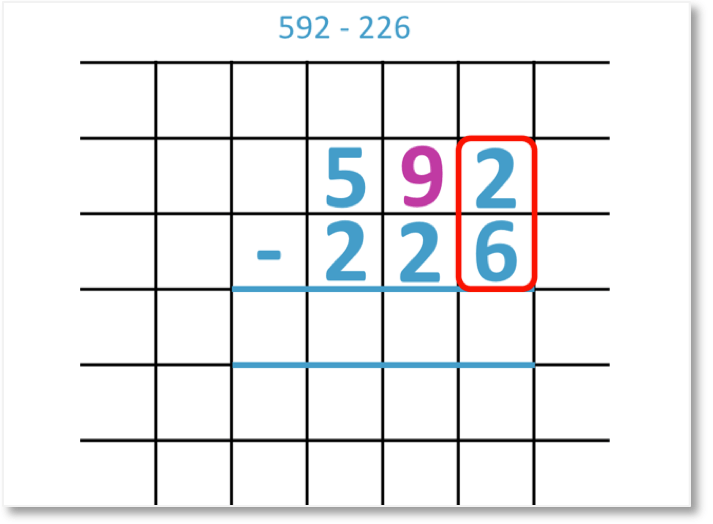We must therefore borrow some units from the tens column. We can borrow from the 9 tens.We regroup 1 ten to borrow and we carry it over into the units column, next to the 2. In total, we now have 12 units. This leaves us with 8 tens in the tens column.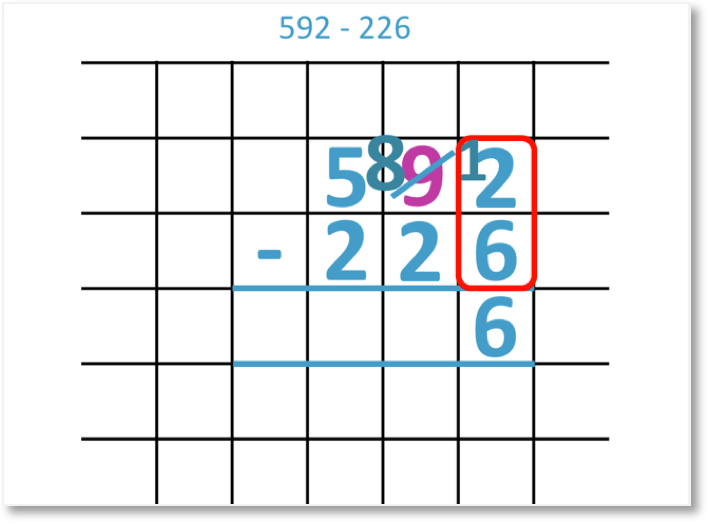Following this regrouping, we can now subtract the units.

12 – 6 = 6

Next, we look at the tens column.Because we borrowed from the 9, we are now left with 8 tens.

8 – 2 = 6

Finally, we look at the hundreds column.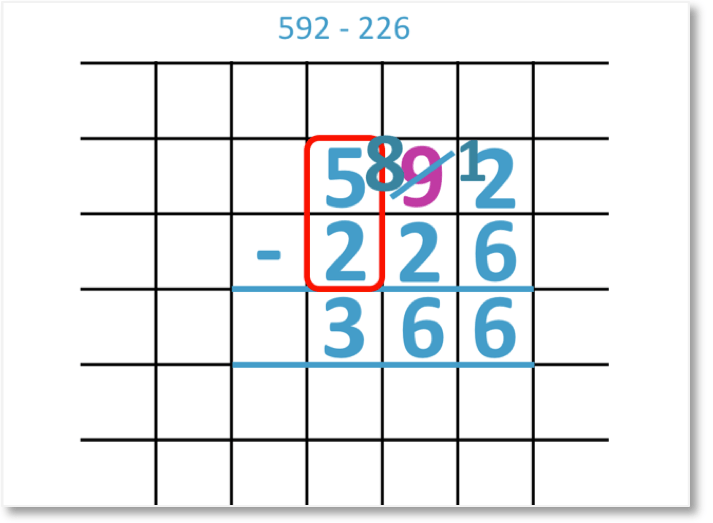5 – 2 = 3

Therefore,

592 – 226 = 366.

Another column subtraction example that we will look at is 674 – 482.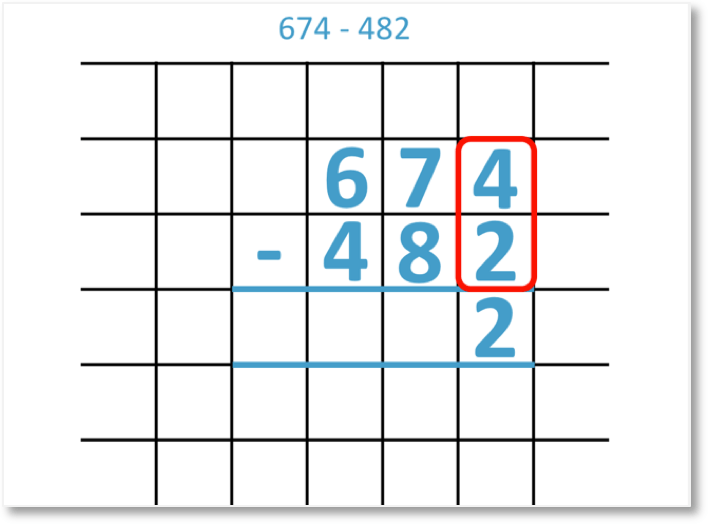Again, we line up the digits of each number according to their place value columns. We start by subtracting the digits in the units column.

4 – 2 = 2

Next, we look at the tens column.The 8 is larger than the 7, so we can’t take it away without regrouping. We must therefore borrow from the 6 in the hundreds column.We regroup 1 hundred for the tens column and write it next to the 7. This gives us 17 in the tens column. We are left with 5 in the hundreds column.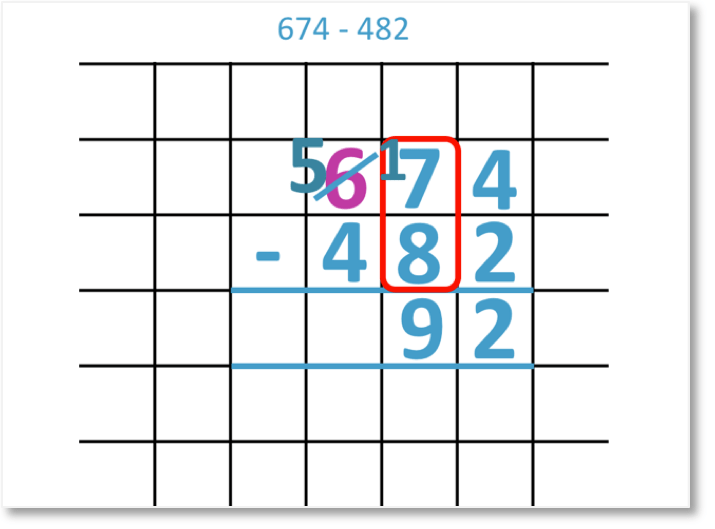Following this borrowing, we can now subtract the tens column.

17 – 8 = 9

Finally, we look at the hundreds column.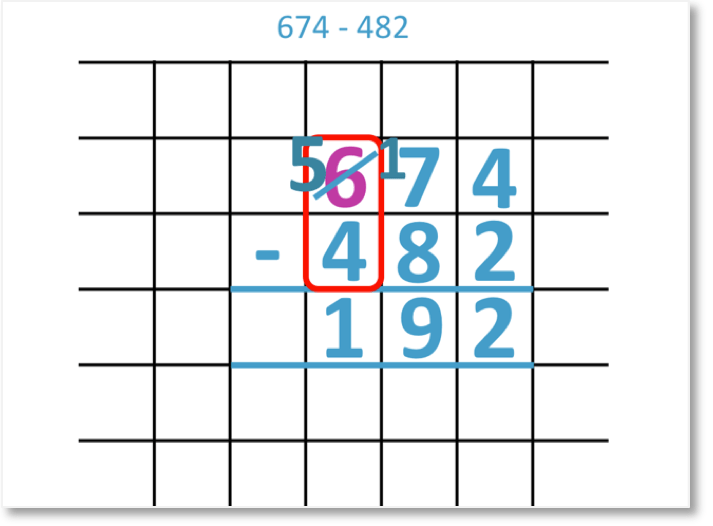Because we borrowed from the 6, we are now left with 5 hundreds.

5 – 4 = 1

Therefore,

674 – 482 = 192.error: Content is protected !!